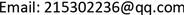1. 引言

DD算法由于计算简洁且容易实现，是迄今最为普及的先验信噪比估计算法。由于该算法中纯净语音谱与噪声谱相互正交的不合理假设，以及采用最大似然估计算法估计当前帧先验信噪比过程中在跟踪后验信噪比时引起一帧的时延，使得该算法音乐噪声较大。针对此缺点，后续有人提出一系列改进算法。其中运行效果较理想的是融合耦合因子算法。该算法在DD算法的基础上引入两个不同平滑参数取值的先验信噪比估计，融入一个耦合参数进行调和，在实际和估计的先验信噪比中建立代价函数求出耦合参数真实值，最终得到新的先验信噪比估计值。该算法有效避免了时延问题，能够实时跟踪信噪比的快速变化，同时失真程度大大降低。但是由于该算法在DD算法估计中采用最大似然法对后验信噪比估计，以此代替当前帧的先验信噪比估计。这使得在无语音活动区产生较大波动，输出的语音信号残留孤立峰值居多，继而产生“音乐噪声”。为了解决上述问题，本文将融合耦合因子算法进行改进，用递归平滑的方式计算后验信噪比，代替传统的瞬时后验信噪比并带入最大似然估计中，有效减少了信号的波动，同时音乐噪声抑制能力有所提升。

2. 语音增强算法的基本理论

y ( t ) = x ( t ) + d ( t ) (1)

Y m , k = X m , k + D m , k (2)

X ^ m , k = G m , k ⋅ Y m , k (3)

G m , k = ξ m , k 1 + ξ m , k (4)

ξ ^ m , k D D = λ ξ ^ m − 1 , k + ( 1 − λ ) ⋅ ξ ^ m , k M L (6)

ξ ^ m , k 1 = a ξ ^ m − 1 , k + ( 1 − a ) ξ ^ m , k M L (7)

ξ ^ m , k 2 = b ξ ^ m − 1 , k + ( 1 − b ) ξ ^ m , k M L (8)

ξ ^ m , k C C = μ ξ ^ m , k 1 + ( 1 − μ ) ξ ^ m , k 2 = ( μ a − μ b + b ) ξ ^ m − 1 , k + ( 1 − μ a + μ b − b ) ξ ^ m , k M L (9)

J = E { ( ξ ^ m , k C C − ξ m , k ) 2 } (10)

μ = ( 1 − b ) ( ξ ^ m , k M L + 1 ) 2 − b ( ξ ^ m - 1 , k − ξ ^ m , k M L ) 2 ( a − b ) [ ( ξ ^ m - 1 , k − ξ ^ m , k M L ) 2 + ( ξ ^ m , k M L + 1 ) 2 ] (11)

3. 改进先验信噪比的新型算法

γ ^ m , k = β ⋅ γ ^ m − 1 , k + ( 1 − β ) ⋅ min [ γ m , k , 20 ] (12)

ξ ^ m , k 1 = a ξ ^ m − 1 , k + ( 1 − a ) γ ^ M L m , k (13)

ξ ^ m , k 2 = b ξ ^ m − 1 , k + ( 1 − b ) γ ^ M L m , k (14)

4. 仿真结果比较

The SegSNR data comparison table of the four algorithm

DD算法CC算法改进算法
White0 dB4.39075.80057.1560
5 dB5.84346.63597.8063
10 dB7.09847.73208.6589
15 dB9.198010.81111.2345
Pink0 dB5.15655.28086.6384
5 dB6.19756.22427.4263
10 dB7.57817.64498.6095
15 dB10.255810.772111.338
Buccaneer20 dB4.13434.92116.1662
5 dB5.22565.71496.8133
10 dB6.82147.71398.5504
15 dB8.794910.450510.8979
F160 dB5.26235.39916.7674
5 dB6.42016.50447.7754
10 dB7.86567.93919.0580
15 dB10.536310.979811.6437
M1090 dB6.27306.86038.6496
5 dB7.40827.91769.3838
10 dB8.94229.413510.6136
15 dB11.469212.574113.3508

The STOI data comparison table of the four algorithm

DD算法CC算法改进算法
White0 dB72.235075.913177.5240
5 dB79.858381.792382.8082
10 dB85.442085.776486.5817
15 dB89.798489.614690.4553
Pink0 dB73.086376.533977.2854
5 dB79.353482.402783.0000
10 dB85.982687.178587.8651
15 dB90.388590.428991.0242
Buccaneer20 dB70.840575.076675.7672
5 dB76.985380.999381.5867
10 dB83.568085.194885.3056
15 dB88.435588.837188.8654
F160 dB76.207879.554480.7624
5 dB82.566085.077086.1478
10 dB88.194889.256090.5462
15 dB91.739892.137893.2722
M1090 dB76.291679.193879.9584
5 dB82.058284.772685.9888
10 dB87.973988.056489.5696
15 dB90.316390.372291.5371

The LSD data comparison table of the four algorithm

DD算法CC算法改进算法
White0 dB6.12615.74235.0706
5 dB5.56115.38794.8288
10 dB5.40875.12244.6612
15 dB5.06794.32664.0306
Pink0 dB5.24375.11204.4945
5 dB4.93274.92944.3898
10 dB4.82384.49724.0886
15 dB4.47603.75013.5135
Buccaneer20 dB5.94935.47184.8267
5 dB5.63245.26194.6566
10 dB5.39184.69954.2640
15 dB5.09204.08123.8244
F160 dB4.92314.87054.3207
5 dB4.76594.66504.2287
10 dB4.61714.36964.0078
15 dB4.21483.67633.4605
M1090 dB4.33953.40643.1264
5 dB4.14413.40953.1283
10 dB4.14413.03362.8718
15 dB3.61702.52282.4159

5. 结论